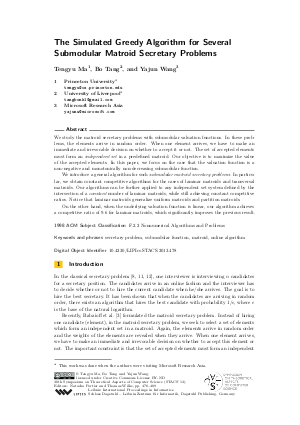Document# The Simulated Greedy Algorithm for Several Submodular Matroid Secretary Problems

### Authors Tengyu Ma, Bo Tang, Yajun Wang## File

LIPIcs.STACS.2013.478.pdf
• Filesize: 0.6 MB
• 12 pages

## Cite As

Tengyu Ma, Bo Tang, and Yajun Wang. The Simulated Greedy Algorithm for Several Submodular Matroid Secretary Problems. In 30th International Symposium on Theoretical Aspects of Computer Science (STACS 2013). Leibniz International Proceedings in Informatics (LIPIcs), Volume 20, pp. 478-489, Schloss Dagstuhl - Leibniz-Zentrum für Informatik (2013)
https://doi.org/10.4230/LIPIcs.STACS.2013.478

## Abstract

We study the matroid secretary problems with submodular valuation functions. In these problems, the elements arrive in random order. When one element arrives, we have to make an immediate and irrevocable decision on whether to accept it or not. The set of accepted elements must form an independent set in a predefined matroid. Our objective is to maximize the value of the accepted elements. In this paper, we focus on the case that the valuation function is a non-negative and monotonically non-decreasing submodular function. We introduce a general algorithm for such submodular matroid secretary problems. In particular, we obtain constant competitive algorithms for the cases of laminar matroids and transversal matroids. Our algorithms can be further applied to any independent set system defined by the intersection of a constant number of laminar matroids, while still achieving constant competitive ratios. Notice that laminar matroids generalize uniform matroids and partition matroids. On the other hand, when the underlying valuation function is linear, our algorithm achieves a competitive ratio of 9.6 for laminar matroids, which significantly improves the previous result.
##### Keywords
• secretary problem
• submodular function
• matroid
• online algorithm

## Metrics

• Access Statistics
• Total Accesses (updated on a weekly basis)
0